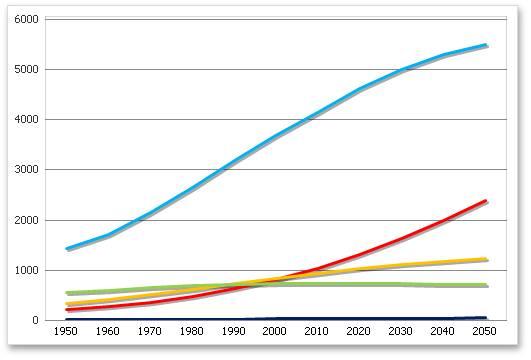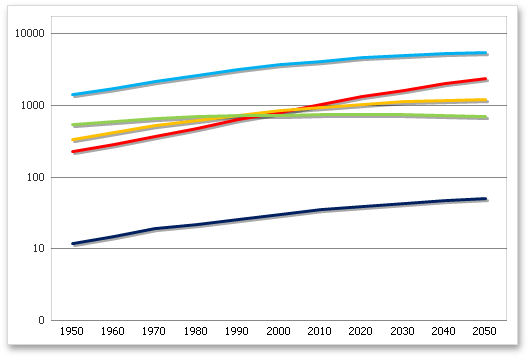.NET Framework 4.5.2+

# AxisScaling.LogScale Property

Gets or sets a value indicating whether the value axis should display its numerical values using a logarithmic scale.

## Declaration

``bool LogScale { get; set; }``

## Property Value

Type Description
Boolean

true, to use the logarithmic axis; otherwise, false.

## Remarks

Set the LogScale property to true to enable the logarithmic display of axis values. In this case, you can define the logarithm base using the AxisScaling.LogBase property.

Usually, the LogScale property is used when the data range of a chart’s series has significant disparity. By enabling this property, the chart’s numerical axis displays all values using logarithmic equivalents. In short, if the logarithmic base is 10, only 5 uniform axis steps will exist between 10 and 1,000,000.

The following table illustrates how the LogScale property works.

LogScale = false

LogScale = true

LogBase = 10## Example

The example below demonstrates how to create a simple line chart and change the value axis to logarithmic using the `AxisScaling.LogScale` property. The logarithmic base, defined by the AxisScaling.LogBase property, is set to 10.

``````Dim worksheet As Worksheet = workbook.Worksheets("chartTask5")
workbook.Worksheets.ActiveWorksheet = worksheet

' Create a chart and specify its location.
Dim chart As Chart = worksheet.Charts.Add(ChartType.Line, worksheet("B2:D8"))
chart.TopLeftCell = worksheet.Cells("F2")
chart.BottomRightCell = worksheet.Cells("L15")

' Set the logarithmic(Log10) type of scale.
chart.PrimaryAxes(1).Scaling.LogScale = True
chart.PrimaryAxes(1).Scaling.LogBase = 10

' Set the position of the legend on the chart.
chart.Legend.Position = LegendPosition.Bottom
``````

The following code snippets (auto-collected from DevExpress Examples) contain references to the LogScale property.

Note

The algorithm used to collect these code examples remains a work in progress. Accordingly, the links and snippets below may produce inaccurate results. If you encounter an issue with code examples below, please use the feedback form on this page to report the issue.